# 在 COMSOL Multiphysics® 中模拟皮尔斯电子枪

2020年 11月 19日

### 电子枪设计

1. 提取足够多的电子，即获得足够的电子束流
2. 将电子加速到一定的动能
3. 将光束聚焦在特定位置

### 两个带电粒子之间的力

\mathbf{F}_1 = \frac{q_1 q_2}
{4\pi\epsilon_0}\frac{\mathbf{r}_1-\mathbf{r}_2}{\left|\mathbf{r}_1-\mathbf{r}_2\right|^3}

### 大量带电粒子之间的力

\mathbf{F}_1 = \sum_{j=2}^N\frac{q_1 q_j}{4\pi\epsilon_0}\frac{\mathbf{r}_1-\mathbf{r}_j}{\left|\mathbf{r}_1-\mathbf{r}_j\right|^3}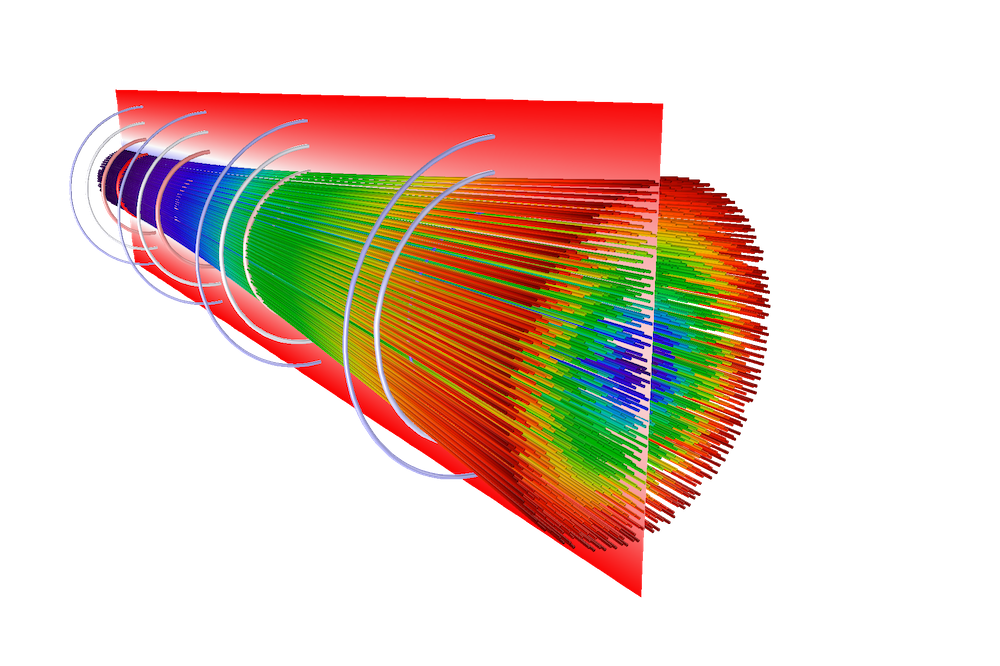### 寻找最佳电极形状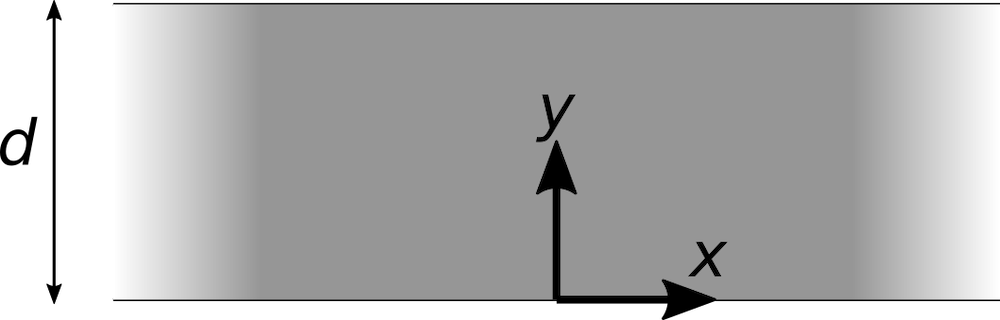V = V_\textrm{a}\left(\frac{y}
{d}\right)^{4/3}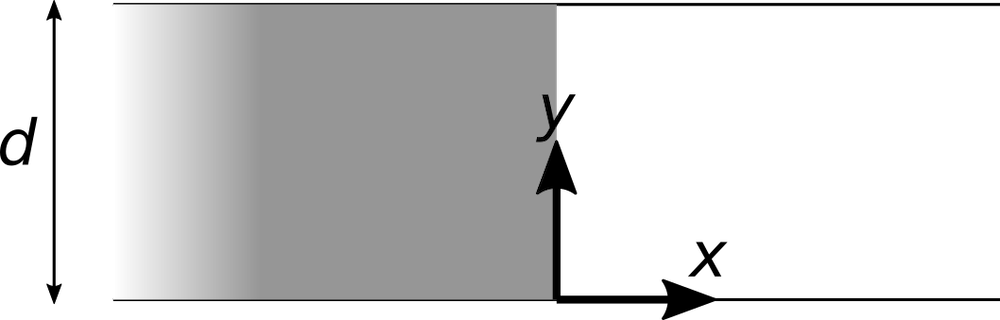### 电子枪设计的皮尔斯方法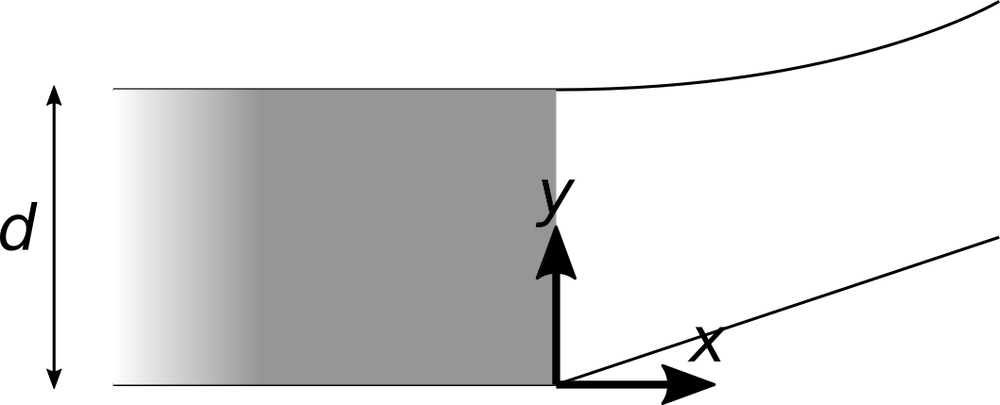\nabla^2 V = 0

\begin{align}
\frac{\partial^2 f}{\partial x^2} + \frac{\partial^2 f}{\partial y^2}
&= \frac{\partial}{\partial x}\left(\frac{\partial u}{\partial x}\frac{\partial f}{\partial u}\right)
+\frac{\partial}{\partial y}\left(\frac{\partial u}{\partial y}\frac{\partial f}{\partial u}\right)\\
&= \frac{\partial}{\partial x}\left(i\frac{\partial f}{\partial u}\right)
+\frac{\partial}{\partial y}\left(\frac{\partial f}{\partial u}\right)\\
&= \frac{\partial u}{\partial x}\frac{\partial}{\partial u}\left(i\frac{\partial f}{\partial u}\right)
+\frac{\partial u}{\partial y}\frac{\partial}{\partial u}\left(\frac{\partial f}{\partial u}\right)\\
&= i^2\frac{\partial^2 f}{\partial u^2} + \frac{\partial^2 f}{\partial u^2}\\
&= -\frac{\partial^2 f}{\partial u^2} + \frac{\partial^2 f}{\partial u^2}\\
&= 0
\end{align}

V = \textrm{Re}\left[f(u)\right]

V = V_\textrm{a}\textrm{Re}\left[\left(\frac{u}{d}
\right)^{4/3}\right]

u=re^{i\theta}

V = V_\textrm{a}\textrm{Re}\left[\left(\frac{r}
{d}e^{i\theta}\right)^{4/3}\right]

V = V_\textrm{a}\left(\frac{r}{d}\right)^{4/3}\textrm{Re}\left(e^{4i\theta/3}\right)

V = V_\textrm{a}\left(\frac{r}{d}
\right)^{4/3}\cos\frac{4\theta}
{3}

V=0 的解是直线

\frac{4\theta}{3}
= \frac{\pi}{2}

V = Va的解是曲线，

r=d\left(\sec\frac{4\theta}{3}\right)^{3/4}

### 创建 COMSOL Multiphysics® 几何### 对粒子场相互作用进行建模

1. 跟踪粒子，而无需考虑束流电子之间的静电排斥。根据此解，估算电子束中的空间电荷密度。
2. 使用估计的空间电荷密度以及阴极和阳极表面的边界条件，计算出静态电势。
3. 使用上一步中的电势来定义粒子上的电力。再次跟踪粒子，并计算电子束中的空间电荷密度。
4. 继续在步骤 2 和 3 之间交替进行固定次数的迭代，或者直到解在迭代之间没有明显变化为止。

### 结果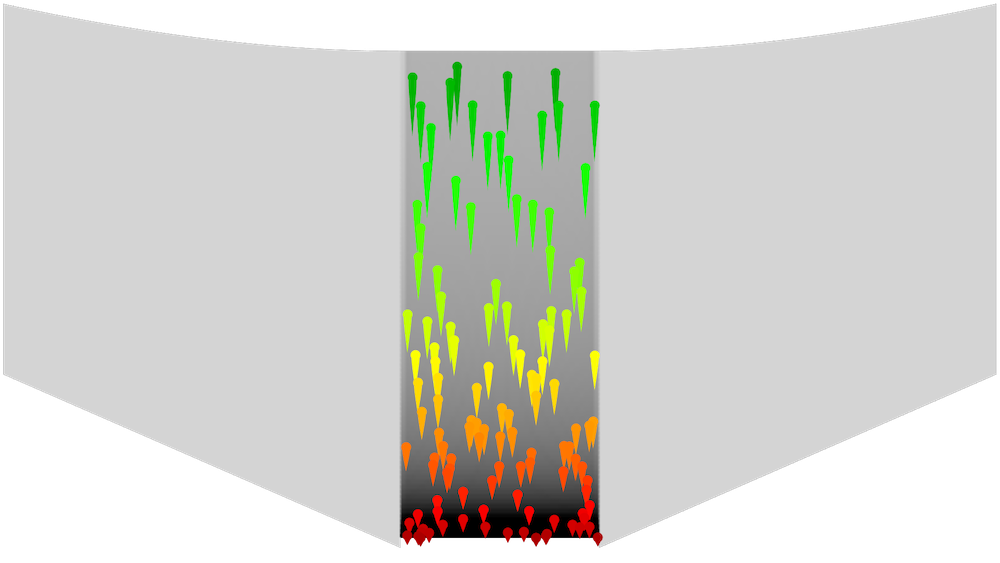### 延伸阅读

Child 定律是基于一个简化的近似，它忽略了释放电子的热速度。实际上，室温下的粒子离开阴极后，可以以每秒几百米的速度四处飞行。要了解有关发射电子的热分布及其对空间电荷受限电子发射（有时称为 Langmuir-Fry 模型（参考文献4，5））的影响的更多信息，请参见平面二极管中的热电子发射示例

1. S. Humphries, Stanley,Charged Particle Beams, Dover, 2013.
2. J.R. Pierce, Rectilinear electron flow in beamsJournal of Applied Physics, vol. 11, no. 8 pp. 548–554, 1940.
3. C.D. Child, “Discharge from hot CaO”, Physical Review (Series I), vol. 32, no. 5, pp. 492–511, 1911.
4. T.C. Fry, “The thermionic current between parallel plane electrodes; velocities of emission distributed according to Maxwell’s law”, Physical Review, vol. 17, no. 4, pp. 441–452, 1921.
5. I. Langmuir,“The effect of space charge and initial velocities on the potential distribution and thermionic current between parallel plane electrodes”,Physical Review, vol. 21, no. 4,pp. 419–435, 1923.,